## Volume

The volume of a solid body is the amount of space'' it occupies. Volume has units of Length cubed (i.e., cm3, m3, in3, etc.) For example, the volume of a box (Rectangular Parallelepiped) of Length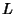, Width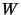, and Heightis given by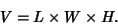The volume can also be computed for irregularly-shaped and curved solids such as the Cylinder and Cube. The volume of a Surface of Revolution is particularly simple to compute due to its symmetry.

The following table gives volumes for some common Surfaces. Heredenotes the Radius,the height,the base Area, and, in the case of the Torus,the distance from the torus center to the center of the tube (Beyer 1987).

 Surface Volume ConeConical FrustumCube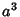CylinderEllipsoid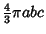Oblate SpheroidProlate Spheroid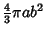Pyramid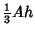Pyramidal Frustum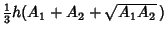Sphere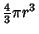Spherical CapSpherical SectorSpherical Segment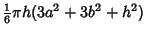TorusEven simple Surfaces can display surprisingly counterintuitive properties. For instance, the Surface of Revolution ofaround the-axis for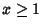is called Gabriel's Horn, and has finite volume, but infinite Surface Area.

The generalization of volume toDimensions foris known as Content.

See also Arc Length, Area, Content, Height, Length (Size), Surface Area, Surface of Revolution, Volume Element, Width (Size)

References

Beyer, W. H. CRC Standard Mathematical Tables, 28th ed. Boca Raton, FL: CRC Press, pp. 127-132, 1987.